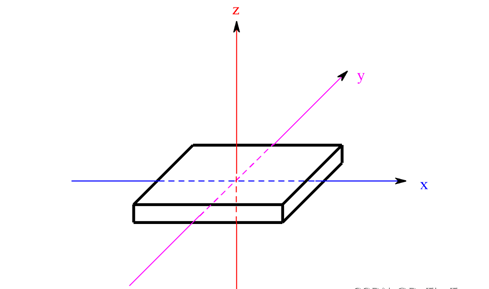Application

# Application of Six-axis Gyroscope in Smart Car

The gyroscope is a sensor that senses the spatial attitude, and is the core element for controlling the balance of the car, judging and adjusting the attitude.

The six-axis gyroscope combines a three-axis gyroscope and a three-axis accelerometer. Its “six axes” are the acceleration xyz axis and the angular velocity xyz axis, which can sense both the angle change and the acceleration change. These six quantities are calculated after calculation., which can return three attitude angles:

Pitch angle (the tilt angle of the front of the car around the Y axis),

The heading angle (the rotation angle of the body around the Z axis),

Roll angle (the angle the side wheel lifts around the X axis).

The main functions of the gyroscope in the racing smart car are as follows:

1. It is used in cascade with the steering ring, and is related to the angular velocity of the Z axis to increase the steering force;
2. Adjust the posture of the balance car so that the body is balanced and can turn and move forward normally
3. The input of the angle loop closed-loop control, such as crossing the three-pronged road to hit a 60-degree angle;
4. Determine the body posture and adjust the state. For example, I have sensed the angle change of the roundabout; sensed the rollover and protected it;
5. Temperature sensor, motor high temperature alarm (the gyroscope is generally close to the motor and has its own temperature sensor)

. Basic usageA six-axis gyroscope combines a three-axis angular velocity and a three-axis accelerometer.

1. Angular Velocity Meter

The data returned by the angular velocity meter is actually the angular velocity in radians. When the gyroscope is rotated, the angular velocity reference value of the corresponding axis is returned. Generally, we need to integrate the angular velocity to obtain the angle, which is intuitive and convenient to use. The angle obtained by the integration is the relative angle, and the attitude at the beginning of the integration is the reference 0 angle.

Integration always introduces error, which increases over time. In addition, the gyroscope also has a zero drift phenomenon, that is, when the car is stationary, it will output a non-zero angular velocity value. The offset value needs to be recalibrated every time the power is turned on, or it needs to be calibrated regularly. Subsequent detection can be eliminated by subtracting the offset value. this error.

1. Accelerometer

The acceleration returned by the accelerometer is not an acceleration in the general sense. The accelerometer is equivalent to a spring system with a weight in the middle and springs in all directions supporting it. When laying flat on the table, some springs are stretched and some are flattened. When changing, different springs are compressed differently, thereby detecting forces in different directions. So actually standing still also produces an “acceleration” value due to gravity. Note that this “acceleration” is not stable, because the acceleration generated by the swing of the car model itself will generate a large interference signal, so it is not appropriate to rely too much on this value.

Only the accelerometer can be used to get the pitch angle of the body, and it is the absolute angle to the ground. In the swing accelerometer, the gravitational acceleration component of the corresponding axis changes, and the change of the angle can be obtained by using the inverse trigonometric function.

gyroscope

accelerometer

mems gyroscope

accelerometer sensor

gyroscope price

types of accelerometer

gyro car

accelerometer price

gyro sensors

micro accelerometer